# 9.6.2. Inverting MT Data¶

Here, we invert synthetic impedance tensor data using E3DMT versions 1 and 2. When inverting impedances, the E3DMT codes have a tendency to place conductive artifacts proximal to the receivers. To overcome this obstacle, we demonstrate a basic approach for limiting artifacts through the use of interface weights.

Important

The MT data are in GIF format; see GIF data conventions page. This means the locations are Easting-Northing-Elevation. The data convention however is defined X = Northing, Y = Easting and Z = Down. Failure to recognize this will cause incorrect interpretation of the data. To get addition background information on the coordinate systems used by the E3DMT codes, see the theory sections of the version 1 and version 2 manuals.

## 9.6.2.1. Setup for the Exercise¶

If you have completed the tutorial “Importing, Interpreting and Preparing NSEM Data”:

If you have NOT completed the previous tutorial and would like to start here, complete the following steps:

Pro tip: To avoid confusion between location and data coordinate systems, use the set data headers tool to define location columns as Easting, Northing and Elevation.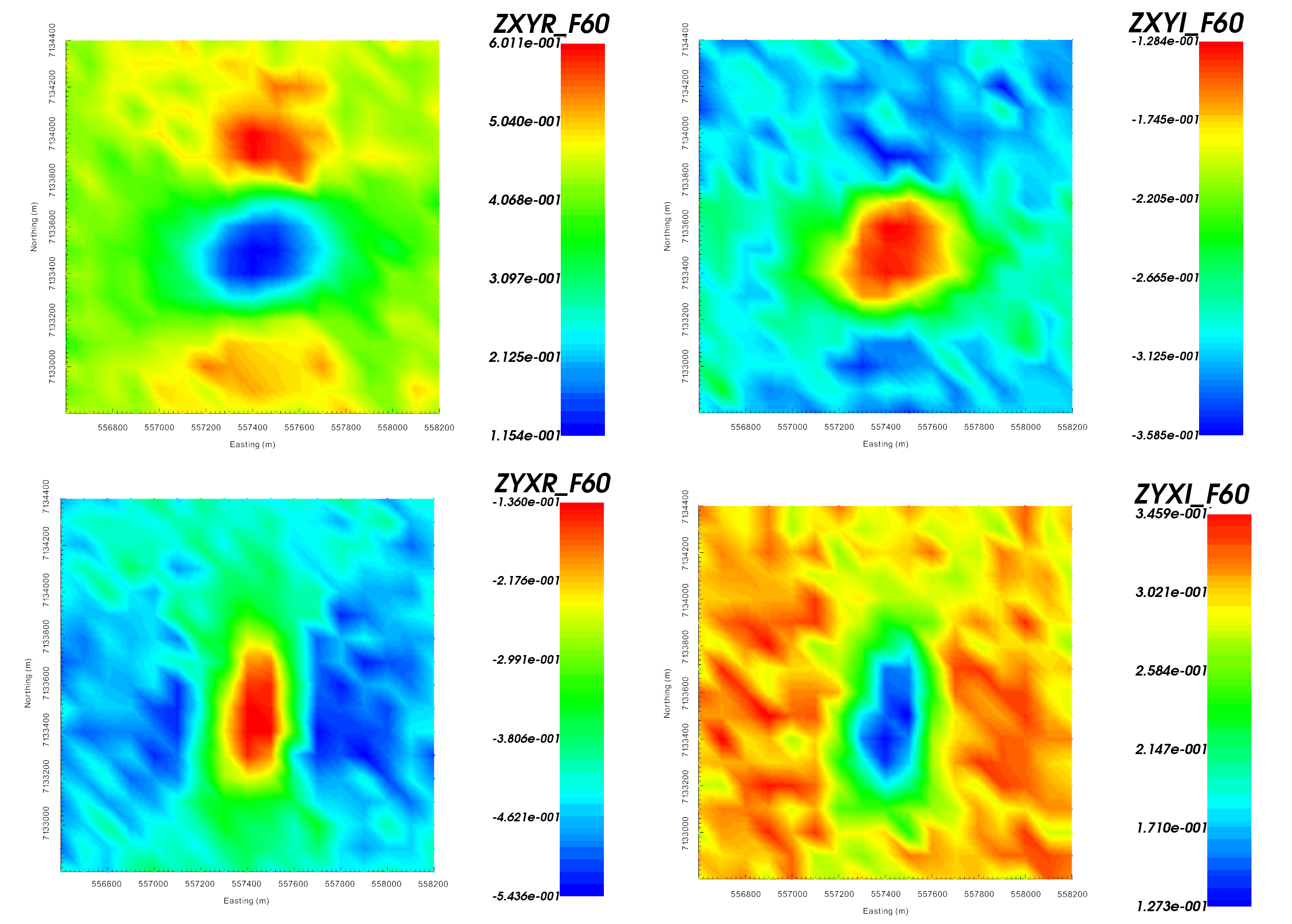Real (left) and imaginary (right) components of impedance tensor element $$Z_{xy}$$ at 60 Hz in V/A. Data shows that $$Z_{xy}$$ lies in the lower-righthand quadrant of the complext plane. This is consistent with the desired format in GIFtools.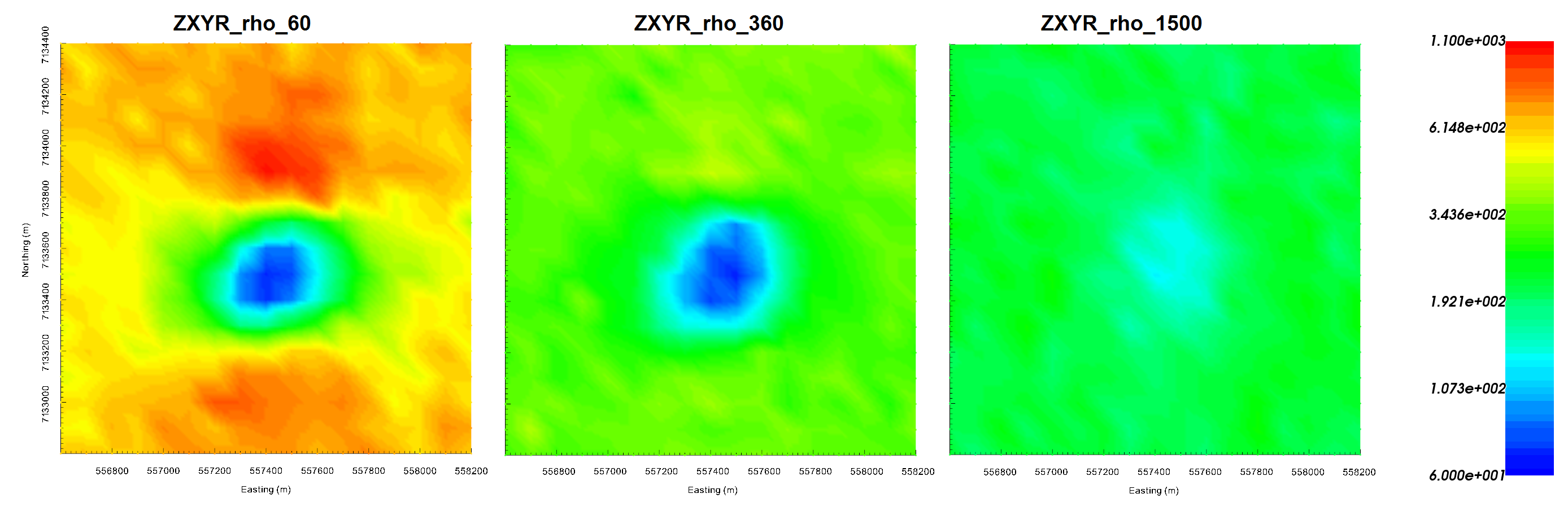Apparent resistivities for $$Z_{xy}$$ at frequencies 60 Hz (left), 360 Hz (middle) and 1500 Hz (right). Apparent resistivity data shows the anomaly as being cause by a conductor.

Important

Data were generated using E3DMT version 2 and a block model approximating TKC. To keep things simple, a constant topography of 400 m. Uncertainties of 0.0025 $$\pm$$ 5% were added to all impedance tensor measurements.

## 9.6.2.2. Reducing Artifacts through Interface Weighting¶

When inverting MT data, the E3DMT codes have a tendency to place conductive structures near receiver locations due to the sensitivity of the data to those cells. Here, we generate interface weights to counteract this problem. By forcing lateral smoothness within the top few layers of cells, we can limit the artifacts and force the inversion to place conductive structures at the appropriate depths.

## 9.6.2.3. E3DMT Version 1¶

Let us now invert the impedance tensor data using E3DMT version 1.

• Create E3DMT version 1 inversion object

• Use edit options to set the inversion parameters

• Basic Tab:
• Select the impedance data

• Set mesh

• Set topography to active cells model

• No background susceptibility

• 1D conductivity of 0.001 S/m (which we inferred from apparent resistivity maps)

• Use Iterative solver unless you have sufficient RAM to use Direct solver.

• Model Options Tab:
• Set Beta cooling schedule to ‘custom by clicking button’. Use beta max = 1, beta min = 1e-8 and reduction factor = 0.25

• Set Chi Factor = 0.8 since E3DMT version 1 uses a non-standard measure of data misfit; see manual <https://e3dmt.readthedocs.io/en/e3dmt/content/theory.html#data-misfit>

• alpha S = 1e-10, alpha E = 1, alpha N = 1 and alpha Z = 2.56 (to recover smoothest model and balance gradient terms based on cell dimensions)

• Set the active cells topo as the active model cells

• Set initial model as 0.001 S/m

• Set reference model as 0.001 S/m

• Set role in model objective function to SMOOTH_MOD_DIF

• Click Apply and write files

• Run the inversion

• View convergence

The results of the inversion are shown below. We are able to recover conductive pipe and the moderately conductive overburden. The conductivty of the overburden is also recovered ($$\sigma \approx 0.005$$ S/m). We are also able to recover the approximate depth to the pipe (~100 m) and the pipe’s general shape.

The inversion reaches target misfit after 3 iterations. The model norm is discontinuous because the current model is set to be the reference model for the next beta value. The recovered model is able to reproduce the observed anomaly quite well. And misfit map indicated we are fitting each component of the data evenly.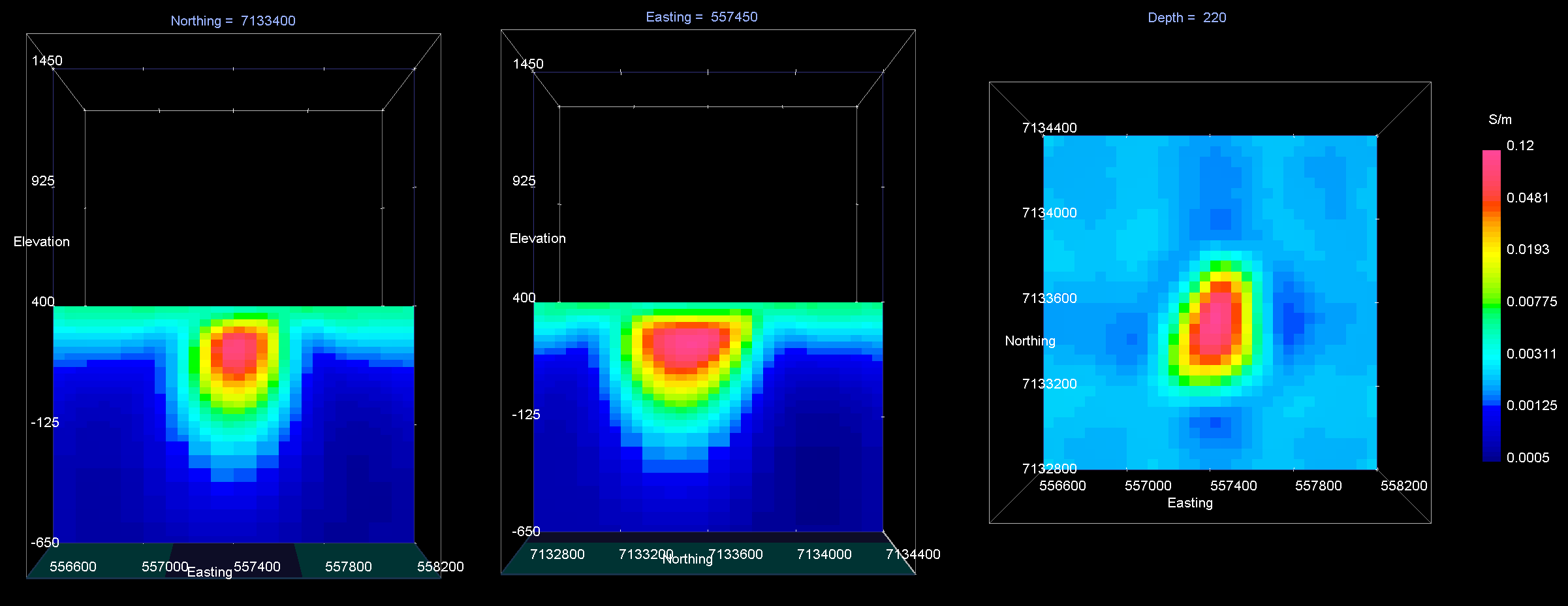Recovered model at iteration 3. EW cross-section (left), NS cross-section (centre), horizontal slice (right).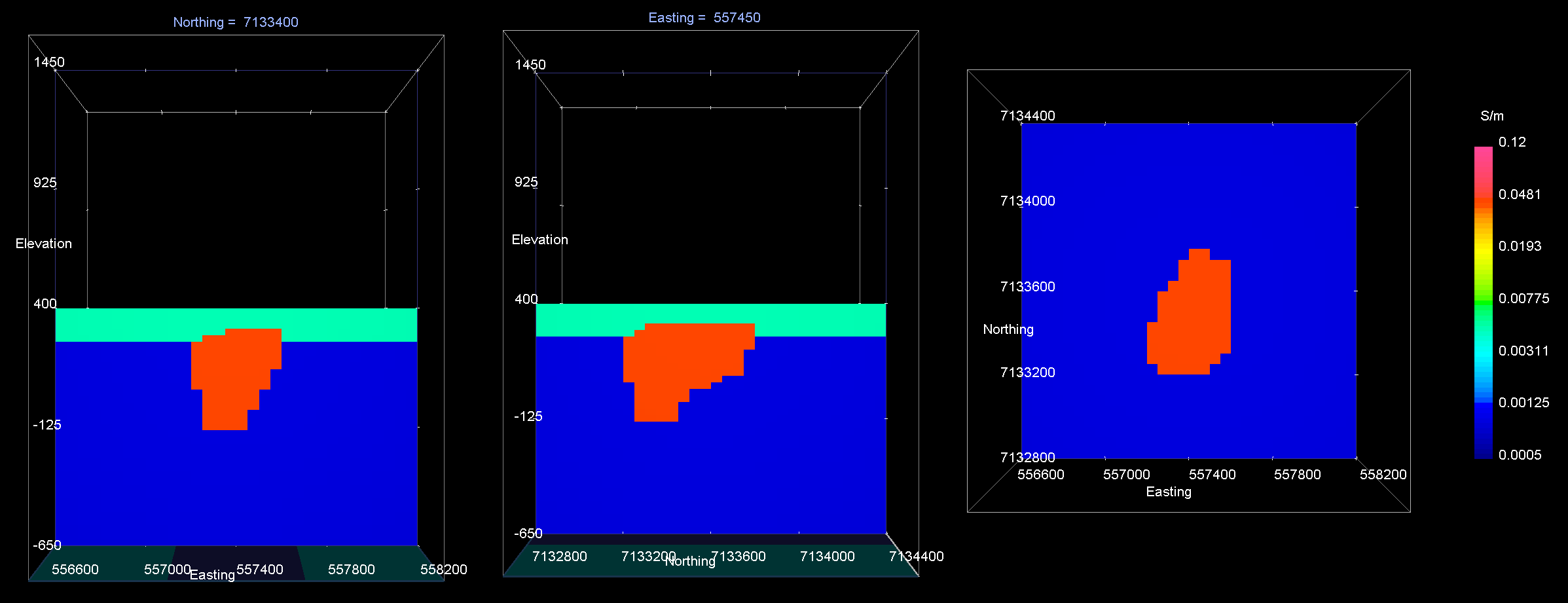True model. EW cross-section (left), NS cross-section (centre), horizontal slice (right).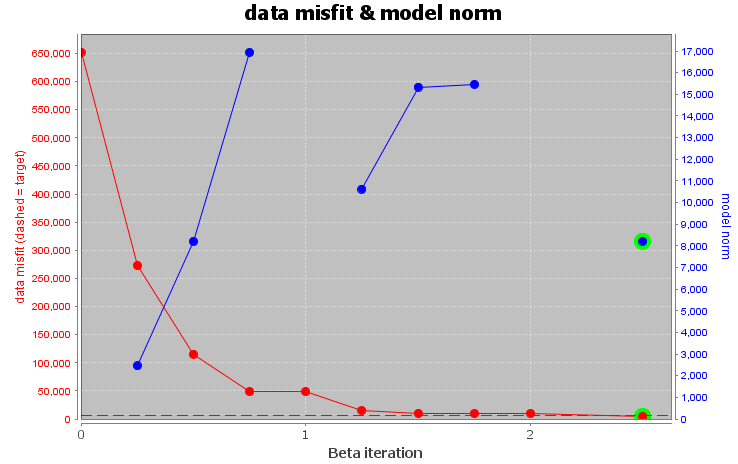Convergence curve shows that inversion reaches target misfit. The model norm is discontinuous because the reference modeled is updated at each iteration.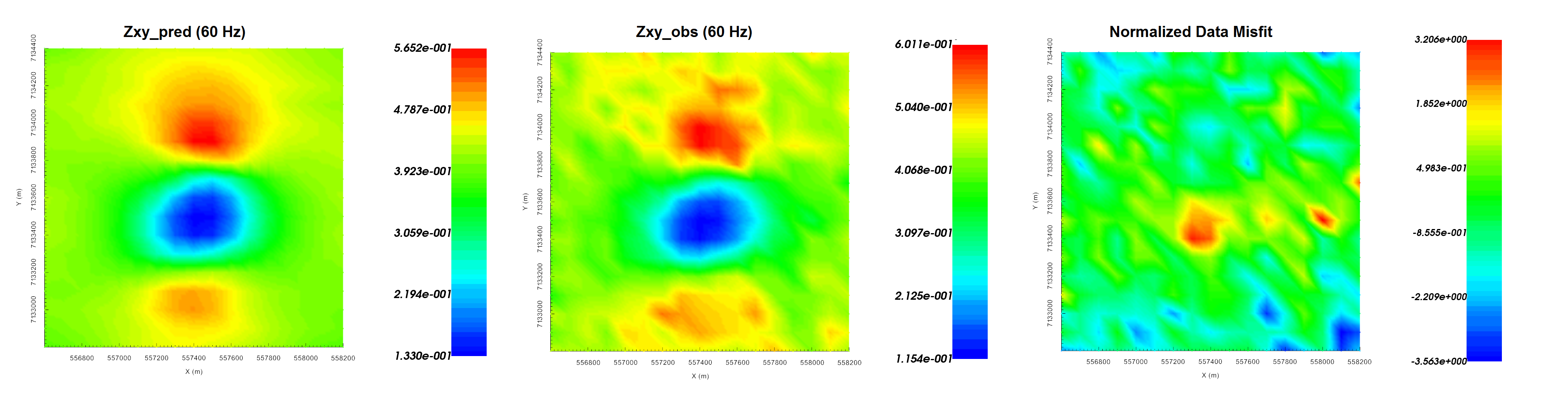Predicted data (left), observed data (centre) and normalized misfit (right) for the real component of $$Z_{xy}$$ at 60 Hz.

## 9.6.2.4. E3DMT Version 2¶

Let us now invert the impedance tensor data using E3DMT version 2. Unlike version 1, version 2 requires that user define the receiver which measure the fields.

• Click the impedance data object and set receivers from locations. Use the following values:

• Easting width = 2 m

• Northing width = 2 m

• Vertical width = 2 m

• Dipole length = 10 m

• Create E3DMT version 2 inversion object

• Use edit options to set the inversion parameters

• Basic Tab:
• Select the impedance data

• Set mesh

• Set topography to active cells model

• No background susceptibility

• 1D conductivity of 0.001 S/m (which we inferred from apparent resistivity maps)

• Use Iterative solver unless you have sufficient RAM to use Direct solver.

• Model Options Tab:
• Set Beta cooling schedule to ‘custom by clicking button’. Use beta max = 1, beta min = 1e-8 and reduction factor = 0.25

• Set Chi Factor = 1

• alpha S = 1e-10, alpha E = 1, alpha N = 1 and alpha Z = 2.56 (to recover smoothest model and balance gradient terms based on cell dimensions)

• Set the active cells topo as the active model cells

• Set initial model as 0.001 S/m

• Set reference model as 0.001 S/m

• Set role in model objective function to SMOOTH_MOD_DIF

• Click Apply and write files

• Run the inversion

• View convergence

The results of the inversion are shown below. We are able to recover conductive pipe and the moderately conductive overburden. The conductivty of the overburden is also recovered ($$\sigma \approx 0.005$$ S/m). We are also able to recover the approximate depth to the pipe (~100 m) and the pipe’s general shape.

The inversion reaches target misfit after 3 iterations. The model norm is discontinuous because the current model is set to be the reference model for the next beta value. The recovered model is able to reproduce the observed anomaly quite well. And misfit map indicated we are fitting each component of the data evenly.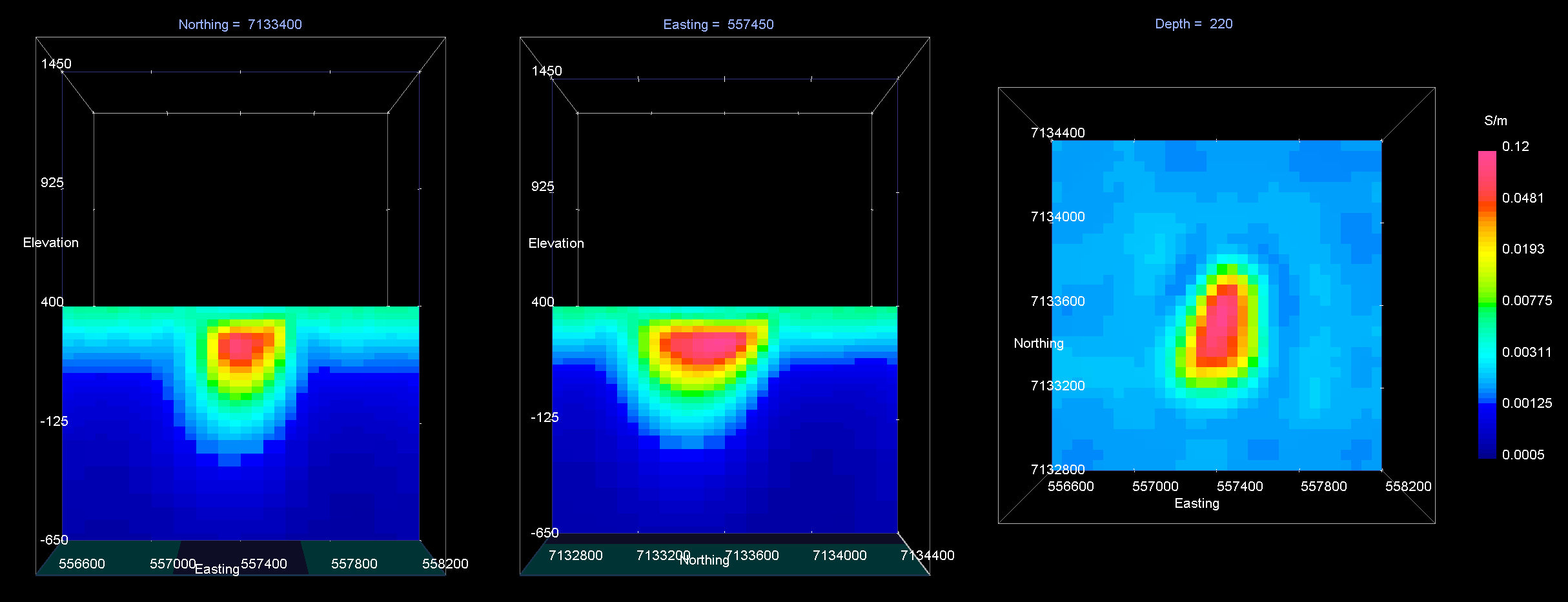Recovered model at iteration 3. EW cross-section (left), NS cross-section (centre), horizontal slice (right).True model. EW cross-section (left), NS cross-section (centre), horizontal slice (right).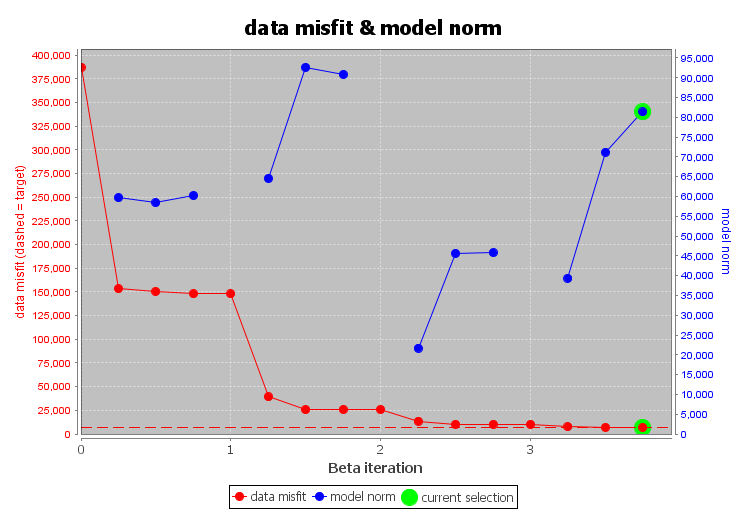Convergence curve shows that inversion reaches target misfit. The model norm is discontinuous because the reference modeled is updated at each iteration.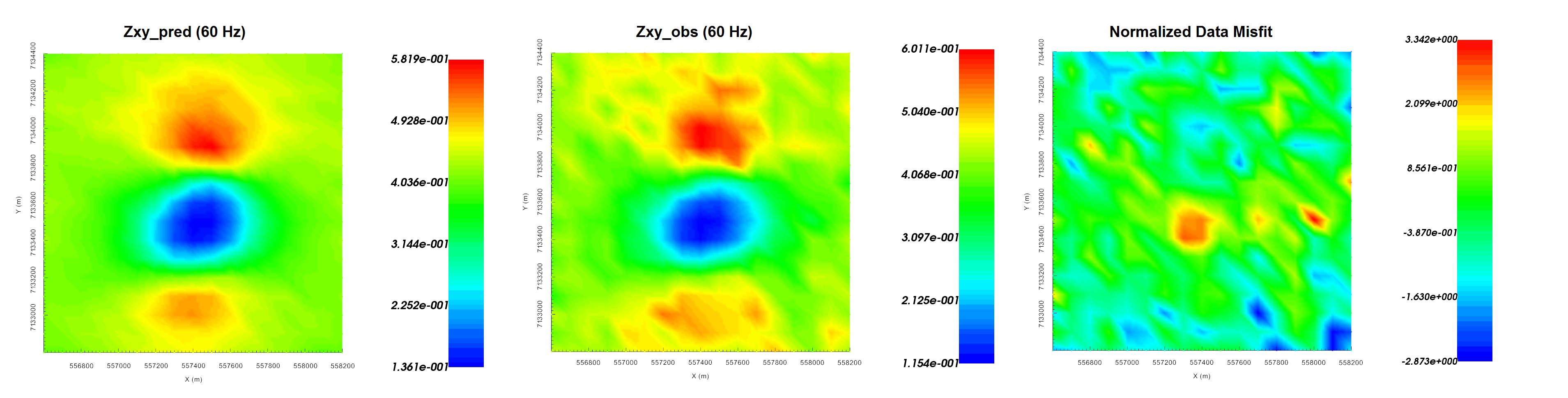Predicted data (left), observed data (centre) and normalized misfit (right) for the real component of $$Z_{xy}$$ at 60 Hz.